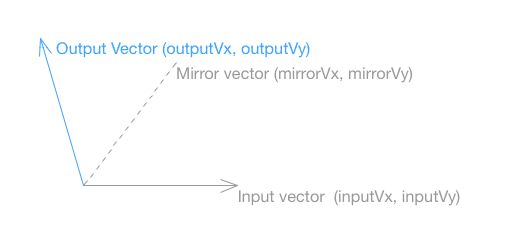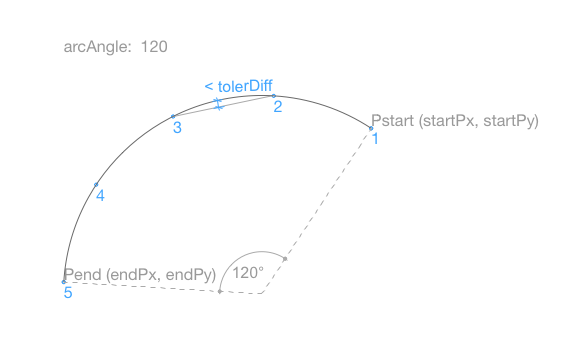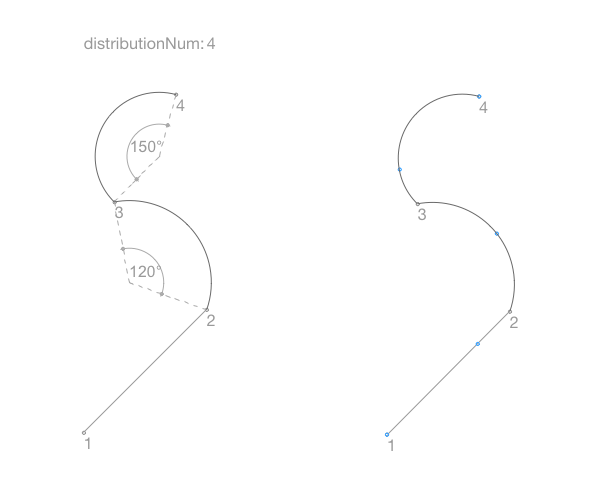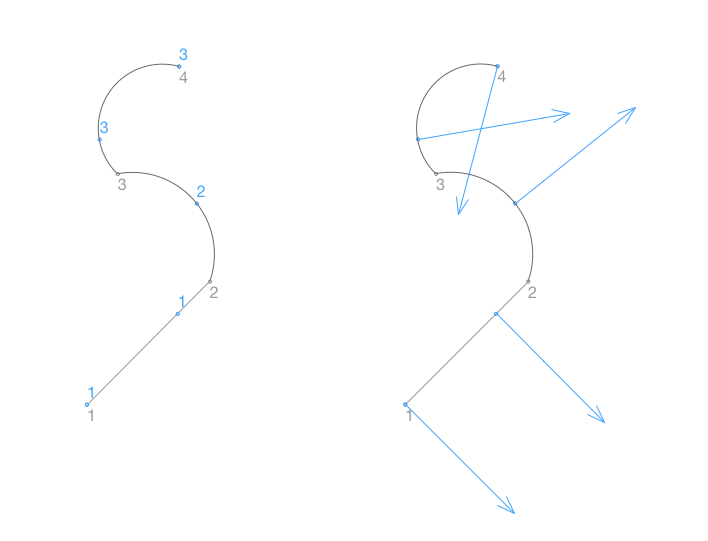## Using BasicGeometricCalc macroExample object for this post

From ARCHICAD 21 a new macro object is available in the ARCHICAD Library: the BasicGeometricCalc macro. As its name suggests, this macro contains basic geometrical calculations, which can come in handy for GDL 2D and 3D scripting. In the normal, international standard-based ARCHICAD Libraries this macro is under the following path: ArchiCAD Library 21/Object Library 21/3. MACROS 21/Base Macros 21/BasicGeometricCalc.gsm. Feel free to save a copy of this macro with a different name, and use it in your own library packages. If any problem or question occurs regarding this macro, please notify us in a comment under this post.

The BasicGeometricCalc macro only uses the Master script and it can be called from 2D scripts and 3D scripts as well.

The macro can handle more types of geometric calculations. The required method should be given in the macro call with the input data belonging to it. The result of the calculation is given back as returned parameters from the macro call. Note that the returned vectors are always unit vectors (their length is 1 m), and as an input vector the macro expects unit vectors as well.

This post introduces the available calculation methods of the macro, directed by the iFunction parameter. The values of this parameter are referred by named variables in the BasicGeometricCalc macro and in this post as well:

```DIRECTION_POINTS		= 1
MIRROR_VECTOR			= 2
DIRECTION_SEGMENT		= 3
INTERSECT_LINE_LINE		= 4
INTERSECT_CIRCLE_LINE		= 5
INTERSECT_SEGMENT_LINE		= 6
INTERSECT_POLY_LINE		= 7
INSERT_POINT_TO_SEGMENT		= 8
SEGMENT_ARC			= 9
INTERSECT_CIRCLE_CIRCLE		= 10
INTERSECT_SEGMENT_CIRCLE	= 11
DISTRIBUTION_POLY_LINE		= 12```

### Direction vector of two Points

Given two points in the space (Point1x, Point1y, Point1z and Point2x, Point2y, Point2z) it returns the direction vector of the line aligned on the given points (DirectionVx, DirectionVy, DirectionVz) and the distance between them (Distance). The direction vector is a unit vector and it points from the second given point to the first point.

```call "BasicGeometricCalc" parameters 	iFunction 	= DIRECTION_POINTS,
Point1x		= Point1x,
Point1y		= Point1y,
Point1z		= Point1z,
Point2x		= Point2x,
Point2y		= Point2y,
Point2z		= Point2z,
returned_parameters	DirectionVx,
DirectionVy,
DirectionVz,
Distance
```### Vector mirrored across an axis

Given two vectors on the x-y plane it returns the direction of the second given vector (inputVx, inputVy) mirrored across the first one (mirrorVx, mirrorVy). The mirroring is executed in a 2D space, the input vectors can be given by two coordinates, and have to be unit vectors.

```call "BasicGeometricCalc" parameters	iFunction 	= MIRROR_VECTOR,
mirrorVx	= mirrorVx,
mirrorVy	= mirrorVy,
inputVx		= inputVx,
inputVy		= inputVy,
returned_parameters	outputVx,
outputVy
```### Direction vectors of segment end points

Given a segment on the x-y plane it returns the two direction vectors pointing from the segment end points to the midpoint of the segment.

The segment can be arched, defined by the angle of the arc (arcAngle). In this case the centerpoint coordinates of the arc are returned, the direction vectors are tangential. The direction of the arc depends on the sign of the angle parameter. In case of straight segment the centerpoint coordinates are `centerArcPx = 0, centerArcPy = 0`.

```call "BasicGeometricCalc" parameters	iFunction	= DIRECTION_SEGMENT,
startPx		= startPx,
startPy		= startPy,
endPx		= endPx,
endPy		= endPy,
arcAngle	= arcAngle,
returned_parameters	StartDirectionVx,
StartDirectionVy,
EndDirectionVx,
EndDirectionVy,
centerArcPx,
centerArcPy
```

### Intersection of two lines

Given two lines on the x-y plane, each line by a point and a direction vector, this function returns whether the two line have an intersection point, and if they do, the coordinates of this point.
If the two given lines are parallel, the value of the state is 0, otherwise it is 1.

```call "BasicGeometricCalc" parameters	iFunction	= INTERSECT_LINE_LINE,
vx1		= vx1,
vy1		= vy1,
px1		= px1,
py1		= py1,
vx2		= vx2,
vy2		= vy2,
px2		= px2,
py2		= py2,
returned_parameters	ipx,
ipy,
state
```

### Intersection of a circle and a line

Given a line on the x-y plane by a point (linePx, linePy) and a direction vector (lineVx, lineVy) and a circle by its centerpoint coordinates (xo, yo) and radius (ro), this function returns whether the line and a circle have intersection point, and if they do, the coordinates and number of these points (state).

If the given line do not cross the circle, the value of the state is 0. If the line is a tangent of the circle, the state is 1, and the coordinates of the tangential point are ipx1 and ipy1. If the line crosses the circle twice, the value of the state is 2, the coordinates of the intersection points are ipx1, ipy1 and ipx2, ipy2.

```call "BasicGeometricCalc" parameters	iFunction	= INTERSECT_CIRCLE_LINE,
xo		= xo,
yo		= yo,
ro		= ro,
linePx		= linePx,
linePy		= linePy,
lineVx		= lineVx,
lineVy		= linePy,
returned_parameters	ipx1,
ipy1,
ipx2,
ipy2,
state
```

### Intersection of a segment and a line

Given a line on the x-y plane by a point (line_px, line_py) and a direction vector (line_vx, line_vy) and a segment by its two endpoint coordinates (segment_p1x, segment_p1y, segment_p2x, segment_p2y) and its angle (segment_ang), this function returns whether the line and a segment have intersection point, and if they do, the coordinates and number of these points (state).

The segment can be arched, the sign of the angle (segment_ang) defines the arc direction. If the given line do not cross the segment, the value of the state is 0. If the line is a tangential to the segment or crosses it once, the state is 1, and the coordinates of the intersection point are ipx1 and ipy1. If the line crosses the segment twice, the value of the state is 2, the coordinates of the intersection points are ipx1, ipy1 and ipx2, ipy2.

```call "BasicGeometricCalc" parameters	iFunction	= INTERSECT_SEGMENT_LINE,
line_vx		= line_vx,
line_vy		= line_vy,
line_px		= line_px,
line_py		= line_py,
segment_p1x	= segment_p1x,
segment_p1y	= segment_p1y,
segment_p2x	= segment_p2x,
segment_p2y	= segment_p2y,
segment_ang	= segment_ang,
returned_parameters	ipx1,
ipy1,
ipx2,
ipy2,
state
```

### Intersection of a polygon and a line

Given a line on the x-y plane by a point (px, py) and a direction vector (vx, vy) and a polygon by its two coordinates of each points and angle of each segments, this function returns the number of their intersection points, each intersection point’s coordinates and the index of the segment which contains intersection point.

The input polygon data should be given in a two-dimensional length type array, the PolyCoords[]. This array has as many rows as polygon points, and 3 columns for each segment data:

• PolyCoords[i]: Coordinate X of segment start point
• PolyCoords[i]: Coordinate Y of segment start point
• PolyCoords[i]: Angle of segment which starts from segment start point

The polygon points should be given without closing point. The sign of the angle defines the arc direction of the arched segments.

The polygon on the picture below is given by the following input values:

```! 1st Polygon Point input data
PolyCoords = 0
PolyCoords = 0
PolyCoords = 0

! 2nd Polygon Point input data
PolyCoords = 0.8
PolyCoords = 0
PolyCoords = 120

! 3rd Polygon Point input data
PolyCoords = 1
PolyCoords = 0.8
PolyCoords = 0

! 4th Polygon Point input data
PolyCoords = 0
PolyCoords = 0.8
PolyCoords = 0
```

The number of the Intersection points is returned in the nISectPoints. The coordinates and segment index of each intersection point is returned in the IntersectionPoints one-dimensional array, which have `nISectPoints * 3 `elements. The following three data belongs to each j intersection point:

• IntersectionPoints[j * 3 – 2]: Coordinate X of intersection point
• IntersectionPoints[j * 3 – 1]: Coordinate Y of intersection point
• IntersectionPoints[j * 3]: Index of segment which contains the intersection point

The IntersectionPoints array should be defined before the macro call.

```dim IntersectionPoints[]

call "BasicGeometricCalc" parameters	iFunction	= INTERSECT_POLY_LINE,
PolyCoords	= PolyCoords,
vx		= vx,
vy		= vy,
px		= px,
py		= py,
returned_parameters	nISectPoints,
IntersectionPoints
```

### Insert a point onto a segment with given distance

Given a segment on the x-y plane by its endpoint coordinates (insertionToPx, insertionToPy and benchmarkPx, benchmarkPy) and its angle (segmentAngle) , and a distance (insertionDist), this function returns the coordinates (insertedPx, insertedPy) and angle (insertAngle) which belong to the point inserted onto the segment.

The segment can be arched, the sign of the angle defines the arc direction. The insertion distance is measured from the first given point coordinates. If the segment is arched, the distance is measured on the arc, not the chord. The insertAngle is the angle of the insertionToPx, insertionToPy – insertedPx, insertedPy segment.

```call "BasicGeometricCalc" parameters	iFunction	= INSERT_POINT_TO_SEGMENT,
insertionToPx	= insertionToPx,
insertionToPy	= insertionToPy,
benchmarkPx	= benchmarkPx,
benchmarkPy	= benchmarkPy,
segmentAngle	= segmentAngle,
insertionDist	= insertionDist,
returned_parameters	insertedPx,
insertedPy,
insertAngle
```

### Segmentation of an arc with given tolerance

Given an arched segment on the x-y plane and it returns the polyline coordinates of the arc segmented with the given tolerance (tolerDiff).

The direction of the arc depends on the sign of the angle parameter. The polyline coordinates are returned in the insertedPointData one-dimensional array, which have `numberOfPoints * 2` elements. The following two data belongs to each j segmentation point:

• insertedPointData[j * 2 – 1]: Coordinate X of segmentation point
• insertedPointData[j * 2]: Coordinate Y of segmentation point

The insertedPointData array should be defined before the macro call.

dim insertedPointData[]

```call "BasicGeometricCalc" parameters	iFunction	= SEGMENT_ARC,
startPx		= startPx,
startPy		= startPy,
endPx		= endPx,
endPy		= endPy,
arcAngle	= arcAngle,
tolerDiff	= tolerDiff,
returned_parameters	insertedPointData
```### Intersection of two circles

Given two circles on the x-y plane by their centerpoint coordinates and radius it, returns whether the circles have intersection point, and if they do, the coordinates and number of these points (state).

If the circles do not intersect the value of the state is 0. If the circles have one point of tangency, the state is 1, and the coordinates of the tangential point are ipx1 and ipy1. If the circles have two intersection points, the value of the state is 2, the coordinates of the intersection points are ipx1, ipy1 and ipx2, ipy2.

```call "BasicGeometricCalc" parameters	iFunction	= INTERSECT_CIRCLE_CIRCLE,
circleAcx	= circleAcx,
circleAcy	= circleAcy,
circleAr	= circleAr,
circleBcx	= circleBcx,
circleBcy	= circleBcy,
circleBr	= circleBr,
returned_parameters	ipx1,
ipy1,
ipx2,
ipy2,
state
```

### Intersection of a segment and a circle

Given a circle on the x-y plane by its centerpoint coordinates and radius (circleCx, circleCy and circleR) , and a segment by its two endpoint coordinates (segmP1x, segmP1y, segmP2x, segmP2y) and its angle (segmAng), this function returns whether the circle and the segment have intersection point, and if they do, the coordinates and number of these points (state).

The segment can be arched, the sign of the angle (segmAng) defines the arc direction. If the given circle do not cross the segment, the value of the state is 0. If the circle and the segment have one intersection point, the state is 1, and the coordinates of the intersection point are ipx1 and ipy1. If the circle crosses the segment twice, the value of the state is 2, the coordinates of the intersection points are ipx1, ipy1 and ipx2, ipy2.

```call "BasicGeometricCalc" parameters	iFunction	= INTERSECT_SEGMENT_CIRCLE,
circleCx	= circleCx,
circleCy	= circleCy,
circleR		= circleR,
segmP1x		= segmP1x,
segmP1y		= segmP1y,
segmP2x		= segmP2x,
segmP2y		= segmP2y,
segmAng		= segmAng,
returned_parameters	ipx1,
ipy1,
ipx2,
ipy2,
state
```

### Polyline distribution

Given a polyline on the x-y plane by its two coordinates of each points and angle of each segments (PolylineCoords[]) and an integer number, this function divides the whole polyline for as many segments with equal length as it was given in the integer number, and returns each sector point’s coordinates and the polyline direction at the actual sector point by its perpendicular direction vector coordinates.

The input polyline data should be given in a two-dimensional length type array, the PolylineCoords[]. This array has as many rows as polyline points, and 3 columns for each segment data:

• PolylineCoords[i]: Coordinate X of segment start point
• PolylineCoords[i]: Coordinate Y of segment start point
• PolylineCoords[i]: Angle of segment which starts from segment start point

The number of the polyline sector points are returned in the nDistributorPoints parameter. The polyline sector point data are returned in the DistributorPoints one-dimensional array, which have `nDistributorPoints * 5` elements. The following five data belong to each j sector point:

• DistributorPoints[j * 5 – 4]: Coordinate X of sector point
• DistributorPoints[j * 5 – 3]: Coordinate Y of sector point
• DistributorPoints[j * 5 – 2]: Perpendicular vector X of sector point
• DistributorPoints[j * 5 – 1]: Perpendicular vector Y of sector point
• DistributorPoints[j * 5]: index of segment which contains the sector point

The DistributorPoints array should be defined before the macro call.

```dim DistributorPoints[]

call "BasicGeometricCalc" parameters	iFunction			= DISTRIBUTION_POLY_LINE,
PolylineCoords			= PolylineCoords,
offsetDistributionFromStart	= offsetDistributionFromStart,
offsetDistributionFromEnd	= offsetDistributionFromEnd,
distributionNum			= distributionNum,
returned_parameters	nDistributorPoints,
DistributorPoints
```

The given polyline can contain arched segments, the sign of the angle defines the arc direction. On the arcs the length is measured on the arc, not the chord.

The polyline on the picture below is given by the following input values:

```! 1st Polyline Point input data
PolylineCoords = 0
PolylineCoords = 0
PolylineCoords = 0

! 2nd Polyline Point input data
PolylineCoords = 0.8
PolylineCoords = 0.8
PolylineCoords = 120

! 3rd Polyline Point input data
PolylineCoords = 0.2
PolylineCoords = 1.5
PolylineCoords = -150

! 4th Polyline Point input data
PolylineCoords = 0.6
PolylineCoords = 2.2
PolylineCoords = 0
```The index of the segment which contains the sector point belongs to each sector point data. The polyline direction at the sector points are returned by the perpendicular direction vector coordinates.The distribution method can be specified in a range by giving an offset from the first and last input polyline point.